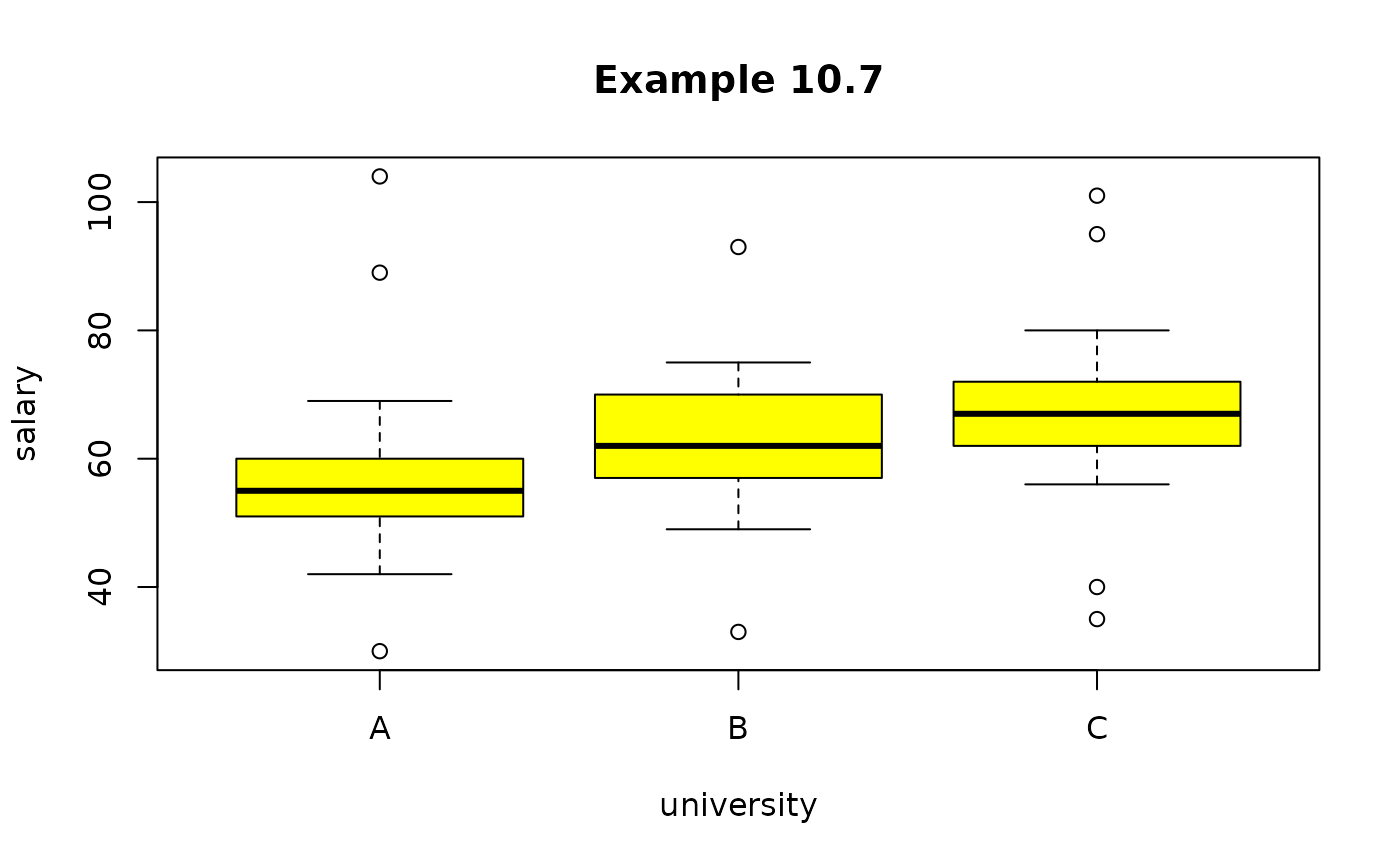Data for Example 10.7

Engineer

## Format

A data frame/tibble with 51 observations on two variables

salary

salary (in \$1000) 10 years after graduation

university

a factor with levels A, B, and C

## Examples


boxplot(salary ~ university, data = Engineer,
main = "Example 10.7", col = "yellow")kruskal.test(salary ~ university, data = Engineer)
#>
#> 	Kruskal-Wallis rank sum test
#>
#> data:  salary by university
#> Kruskal-Wallis chi-squared = 6.3994, df = 2, p-value = 0.04077
#>
anova(lm(salary ~ university, data = Engineer))
#> Analysis of Variance Table
#>
#> Response: salary
#>            Df  Sum Sq Mean Sq F value Pr(>F)
#> university  2   735.2  367.61  1.5272 0.2275
#> Residuals  48 11553.8  240.70
anova(lm(rank(salary) ~ university, data = Engineer))
#> Analysis of Variance Table
#>
#> Response: rank(salary)
#>            Df Sum Sq Mean Sq F value  Pr(>F)
#> university  2 1412.7  706.34  3.5226 0.03737 *
#> Residuals  48 9624.8  200.52
#> ---
#> Signif. codes:  0 ‘***’ 0.001 ‘**’ 0.01 ‘*’ 0.05 ‘.’ 0.1 ‘ ’ 1